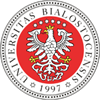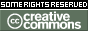REPOZYTORIUM UNIWERSYTETU
W BIAŁYMSTOKUProszę używać tego identyfikatora do cytowań lub wstaw link do tej pozycji: `http://hdl.handle.net/11320/14707`
 Tytuł: Existence and Uniqueness of Algebraic Closures Autorzy: Schwarzweller, Christoph Słowa kluczowe: algebraic closurespolynomial rings with countably infinite numberof variablesEmil Artin Data wydania: 2022 Data dodania: 27-lut-2023 Wydawca: DeGruyter Open Źródło: Formalized Mathematics, Volume 30, Issue 4, Pages 281-294 Abstrakt: This is the second part of a two-part article formalizing existence and uniqueness of algebraic closures, using the Mizar ,  formalism. Our proof follows Artin’s classical one as presented by Lang in . In the first part we proved that for a given field F there exists a field extension E such that every nonconstant polynomial p ∈ F[X] has a root in E. Artin’s proof applies Kronecker’s construction to each polynomial p ∈ F[X]\F simultaneously. To do so we needed the polynomial ring F[X₁, X₂, ...] with infinitely many variables, one for each polynomal p ∈ F[X]\F. The desired field extension E then is F[X₁, X₂, ...]\I, where I is a maximal ideal generated by all nonconstant polynomials p ∈ F[X]. Note, that to show that I is maximal Zorn’s lemma has tobe applied. In this second part this construction is iterated giving an infinite sequence of fields, whose union establishes a field extension A of F, in which every non-constant polynomial p ∈ A[X] has a root. The field of algebraic elements of A then is an algebraic closure of F. To prove uniqueness of algebraic closures, e.g.that two algebraic closures of F are isomorphic over F, the technique of extending monomorphisms is applied: a monomorphism F−→A, where A is an algebraic closure of F can be extended to a monomorphism E−→A, where E is any algebraic extension of F. In case that E is algebraically closed this monomorphism is an isomorphism. Note that the existence of the extended monomorphism again relies on Zorn’s lemma. Afiliacja: Institute of Informatics, University of Gdańsk, Poland URI: http://hdl.handle.net/11320/14707 DOI: 10.2478/forma-2022-0022 ISSN: 1426-2630 e-ISSN: 1898-9934 Typ Dokumentu: Article metadata.dc.rights.uri: https://creativecommons.org/licenses/by-sa/3.0/ Właściciel praw: © 2022 The Author(s)CC BY-SA 3.0 license Występuje w kolekcji(ach): Formalized Mathematics, 2022, Volume 30, Issue 4

Pliki w tej pozycji:
Plik Opis RozmiarFormat
Pozycja ta dostępna jest na podstawie licencji Licencja Creative Commons CCL